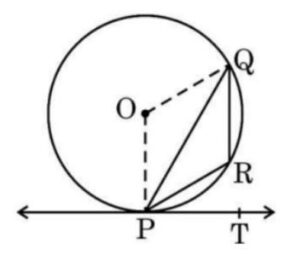Q) In the given figure, PQ is a chord of the circle centered at O. PT is a tangent to the circle at P. If ∠ QPT = 55°, Find the ∠ PRQ.Ans:

Since ∠ OPT = 90° (angle between radius and tangent)

and ∠ QPT = 55°

∴  ∠ OPQ = ∠ OPT –  ∠ QPT  = 90 – 55 = 35°

Next, Since OP = OQ (being radii of the same circle)

∴ ∠ OPQ = ∠ OPQ  (being angles subtended by equal sides)

∴  ∠ OPQ = ∠ OPQ = 35°

Now in Δ QOP,  ∠ OPQ + ∠ OPQ + ∠ QOP = 180° (being angle sum property)

∴  35° + 35° + ∠ QOP = 180°

∴  ∠ QOP = 180° – 35° – 35° = 110°

Since the sum of angle and reflex angle on a point is 360°,

∴  reflex ∠ QOP = 360° – 110° = 250°

Now, since the angle subtended by an arc of  a circle at the centre is 2 times of the angle subtended by it any point on the remaining of the circle:

∴  2 x ∠ PRQ = Reflex ∠ QOP

∴  ∠ PRQ =250° = 125°

Therefore value of ∠ PRQ is 125°

Scroll to Top